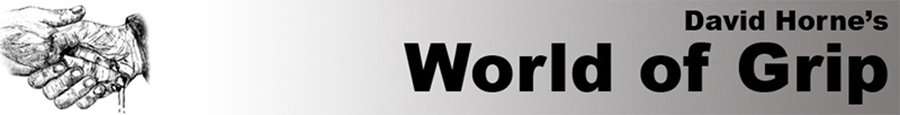athe ultimate online resource for all things grip! aaaaaaaaaaaaaaaaaaaaaaaaaaaaaaaaaawww.davidhorne-gripmaster.com

2005 UK GRIP CONTESTS

Champion of Champions Grip Contest

Saturday 12 November, 2005

Venue: Great Haywood, Staffordshire, England

Promoter: David Horne

All weights in kilo's

Overall Results

1st. David Horne (UK) 569.87
2nd. Nick McKinless (UK/Aust) 557.87
3rd. Steve McGranahan (USA) 537.79
4th. Steve Gardener (UK) 513.97
5th. Frank Henritzi (Ger) 471.89
6th. Jim Wylie (UK) 466.58
7th. Marc Wylie (UK) 453.41
8th. Richard Scott (UK) 419.21
9th. Andrew Christie (UK) 416.46
10th. Dave Johnson (UK) 392.34

1. Two Hands Pinch Lift

1. David Horne 107.2 (WR)
2. Nick McKinless 101.8
3. Steve McGranahan 95.1
4. Frank Henritzi 87.4
5. Steve Gardener 84.5
6. Jim Wylie 75.4
6. Marc Wylie 75.4
8. Richard Scott 72.2
9. Dave Johnson 70.4
9. Andrew Christie 70.4

2. One Hand Vertical Bar Lift

1. Nick McKinless L/H 151.8 R/H 162.9 = 314.7
2. David Horne L/H 141.9 R/H 164.3 (WR) = 306.2
3. Jim Wylie L/H 130 R/H 172.9 (WR) = 302.9
4. Steve Gardener L/H 138.6 R/H 162.9 = 301.5
5. Marc Wylie L/H 151.8 R/H 141.9 = 293.7
6. Andrew Christie L/H 133.3 R/H 133.3 = 266.6
7. Steve McGranahan L/H 130 R/H 133.3 = 263.3
8. Richard Scott L/H 138.6 R/H 123.1 = 261.7
9. Frank Henritzi L/H 128.4 R/H 128.4 = 256.8
10. Dave Johnson L/H 117.2 R/H 130 = 247.2

1. Steve Gardener L/H 110.8 R/H 116.2 = 227
2. David Horne L/H 105.6 R/H 116.2 = 221.8
3. Nick McKinless L/H 105.6 R/H 105.6 = 211.2
4. Steve McGranahan L/H 100.3 R/H 107.9 = 208.2
5. Jim Wylie L/H 80.4 R/H 105.6 = 186
6. Marc Wylie L/H 89.7 R/H 89.7 = 179.4
7. Frank Henritzi L/H 84.5 R/H 89.7 = 174.2
8. Andrew Christie L/H 80.4 R/H 84.5 = 164.9
9. Richard Scott L/H 80.4 R/H 75.2 = 155.6
10. Dave Johnson L/H 75.2 R/H 75.2 = 150.4

4. One Hand Hammer

1. David Horne L/H 9 R/H 9 = 18
2. Steve McGranahan L/H 8.25 R/H 8.5 = 16.75
3. Nick McKinless L/H 7.5 R/H 9 = 16.5
4. Frank Henritzi L/H 6.75 R/H 7.5 = 14.25
5. Jim Wylie L/H 5 R/H 8.5 = 13.5
6. Steve Gardener L/H 5.5 R/H 7.5 = 13
7. Marc Wylie L/H 6 R/H 6.5 = 12.5
8. Richard Scott L/H 5.5 R/H 6.5 = 12
9. Andrew Christie L/H 6 R/H 5.5 = 11.5
10. Dave Johnson L/H 5 R/H 5 = 10

5. One Hand Gripper Hold (weighted strap with 1.25kg)

1. Steve McGranahan L/H 3.09 R/H 3.29 = 6.38
2. Nick McKinless L/H 2.72 R/H 3.29 = 6.01
3. Steve Gardener L/H 2.25 R/H 3.54 = 5.79
4. David Horne L/H 2.72 R/H 2.96 = 5.68
5. Frank Henritzi L/H 2.25 R/H 2.96 = 5.21
6. Jim Wylie L/H 1.73 R/H 2.96 = 4.69
6. Marc Wylie L/H 1.73 R/H 2.96 = 4.69
8. Richard Scott L/H 2.25 R/H 2.25 = 4.50
9. Dave Johnson L/H 2.04 R/H 2.25 = 4.29
9. Andrew Christie L/H 2.04 R/H 2.25 = 4.29

13th British Iron Grip Championships

Saturday 7 May, 2005

Venue: Abbey Life gymnasium, Bournemouth

Promoter: Andrew Christie

All weights in kilo's

Overall Results

1st. Jim Wylie 518.052pts
2nd. Steve Gardener 507.719pts
3rd. Andy Christie 444.933pts
4th. Dave Smith 391.171pts
5th. Chris Williams 382.454pts

1. Grippers

1st Steve Gardener L=2.46 R=3.65 T=6.11
2nd Jim Wylie L=2.46 R=3.29 T=5.75
3rd Andy Christie L=2.25 R=2.76 T=5.01
4th Chris Williams L=2.06 R=2.25 T=4.31
5th Dave Smith L=2.06 R=2.06 T=4.12

2. Two Hands Pinch
Lift

1st Jim Wylie 85k
2nd Steve Gardener 81k
2nd Dave Smith 81k
4th Andy Christie 71k
5th Chris Williams 65k

3. One Hand Vertical Bar Lift

1st Jim Wylie L=140 R=160 T=301.5
2nd Steve Gardener L=132.5 =130 T=264
3rd Andy Christie L=130 R=130 T=261.5
4th Chris Williams L=100 R=100 T= 201.5
5th Dave Smith L=90 R=95 T=186.5

4. One Hand Lift

1st Jim Wylie L=232.5 R=240 T=475.23
2nd Steve Gardener L=220 R=220 T=442.73
3rd Andy Christie L=210 R=210 T=422.73
4th Chris Williams L=210 R=200 T=412.73
5th Dave Smith L=200 R=180 T=382.73

5. Rolling Thunder Lift (Thumbless)

1st Steve Gardener L=100 R=110 T=211.6
2nd Jim Wylie L=90 R=103 T=194.6
3rd Andy Christie L=80 R=80 T=161.6
4th Dave Smith L=65 R=70 T=136.6
5th Chris Williams L=60 R=65 T=126.6